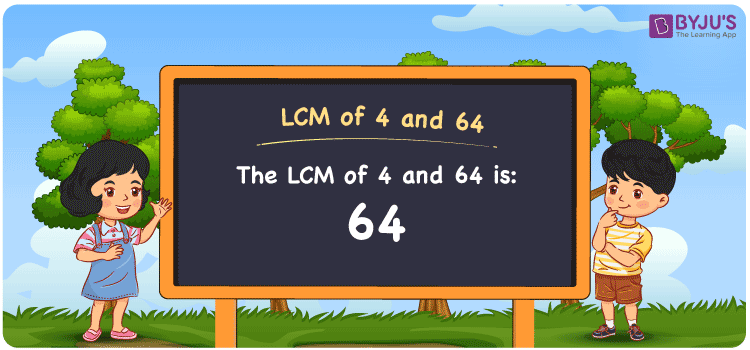# LCM of 4 and 64

LCM of 4 and 64 is 64. The Least Common Multiple or Lowest Common Multiple which is LCM, is the smallest integer that is divisible by the given group of numbers. In the given set of numbers 4 and 64, 64 is the first(least or smallest) number that is common in the set of multiples of 4 and 64. The LCM is also known as LCD, Least Common Divisor for 4 and 64 is 64. You can use the Prime Factorization and Division method to solve the LCMproblems.

## What is LCM of 4 and 64

The Least Common Multiple or Lowest Common Multiple of 4 and 64 is 64.## How to Find LCM of 4 and 64?

LCM of 4 and 64 can be determined using three methods:

• Prime Factorisation
• Division method
• Listing the multiples

### LCM of 4 and 64 Using Prime Factorisation Method

In the Prime Factorisation method, the numbers 4 and 64 can be expressed as;

4 = 2 × 2

64 = 2 × 2 × 2 × 2 × 2 × 2

4 is the common factor of 4 and 64. Along with 4 the other prime factors of 64 are multiplied to get the LCM.

LCM (4, 64) = 2 × 2 × 2 × 2 × 2 × 2 = 64

### LCM of 4 and 64 Using Division Method

In the Division Method, the numbers 4 and 64 are divided together by prime divisors. The common and uncommon prime divisors product forms the LCM.

 2 4 64 2 2 32 2 1 16 2 1 8 2 1 4 2 1 2 × 1 1

Hence, LCM (4, 64) = 2 × 2 × 2 × 2 × 2 × 2 = 64

### LCM of 4 and 64 Using Listing the Multiples

By listing all the multiples of 4 and 64, we can identify the LCM. Below is the list of multiples for 4 and 64.

Multiples of 4

4, 8, 12, 16, 20, 24, 28, 32, 36, 40, 44, 48, 52, 56, 60, 64, 68, 72

Multiples of 64

64, 128, 192

LCM (4, 10) = 64

## Video Lesson on Applications of LCM## Solved Examples

What is the smallest number that is divisible by both 4 and 64?

Answer: 64 is the smallest number that is divisible by both 4 and 64.

What is the LCM for 2, 16, 4 and 64?

Answer: LCM for 2, 16, 4 and 64 is 64 as 2 is the factor for both 4 and 64 whereas 16 is the factor for 64.

## Frequently Asked Questions on LCM of 4 and 64

### What is the LCM of 4 and 64?

The LCM of 4 and 64 is 64.

### Is the LCM of 4 and 64 the same as the HCF of 4 and 64?

No. Least Common Multiple of 4 and 64 is 64 and Highest Common Factor of 4 and 64 is 4.

### What is the common factor of 4 and 64?

1, 2 and 4 are the common factors of 4 and 64.

### What are the first 3 common multiples of 4 and 64?

The first three common multiples of 4 and 64 are 64, 128, and 192.

### Is the LCM of 2 and 4 the same as the LCM of 4 and 64?

No. LCM of 2 and 4 is 4, whereas the LCM of 4 and 64 is 64. They are not the same.